# Compare Ground truth data sets and classes in feature spaces

``````knitr::opts_chunk\$set(warning=FALSE, message=FALSE, fig.height=6, fig.width=8,
fig.path = "figure/grtruthComparison_")

require(raster)
require(rgdal)
require(plyr)
require(scales)
``````

## 1. Data

``````#original digitized polygons
layer="grtruthDN_mean",
stringsAsFactors = FALSE)
grtruthDN_mean@data[1,]
layer="grtruthDN_meanPCA",
stringsAsFactors = FALSE)
grtruthDN_meanPCA@data[1,]
# ground truth by segments (revised: v2)
stringsAsFactors = FALSE)
grthsegMSv2@data[1,]
stringsAsFactors = FALSE)
grthsegMSPCAv2@data[1,]
``````

## 2. Compare segments and original polygons

### 2.1 Compare segments and original polygons: NIR vs RED

``````plot(grtruthDN_mean@data[,c(7,10)],type="n",xlab="RED", ylab="NIR")
text(grtruthDN_mean@data[,c(7,10)],label=grtruthDN_mean@data\$class,
col="red",cex=0.75)
text(grthsegMSv2@data[,c(8,11)],label=grthsegMSv2@data\$class,cex=0.75)
title(main="Ground Truth in NIR vs RED plane",
sub="black:segments; red:digitized polygons")
``````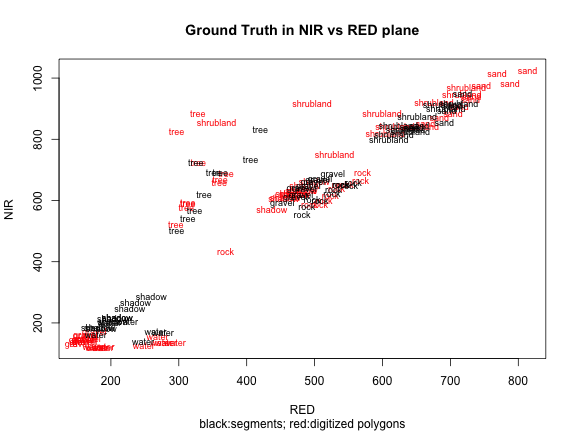Segmentes become “more grey” “shrubland” very close to “sand” errors in water: some “water” segments are actually “shadow”

### 2.2 Compare segments and original polygons: PC2 vs PC1

``````plot(grtruthDN_meanPCA@data[,4:5],type="n")
text(grtruthDN_meanPCA@data[,4:5],labels=grtruthDN_meanPCA@data\$class,
cex=0.75,col="red")
text(grthsegMSPCAv2@data[,5:6],label=grthsegMSPCAv2@data\$class,
cex=0.75)
title(main="Ground Truth in PC space",
sub="black:segments; red:digitized polygons")
``````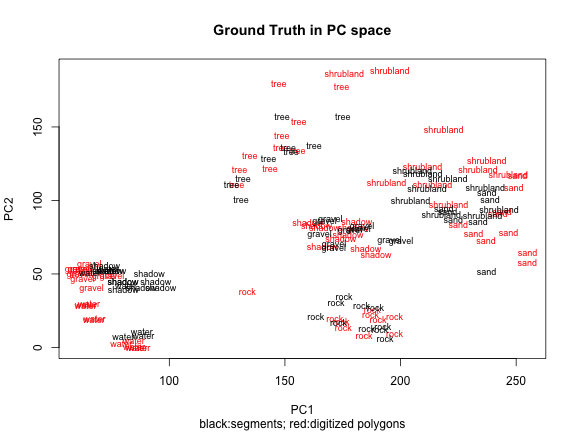``````#labels
plot(grtruthDN_meanPCA@data[,4:5],type="n")
#text(grtruthDN_meanPCA@data[,4:5],labels=grtruthDN_meanPCA@data\$class,
#     cex=0.75,col="red")
text(grthsegMSPCAv2@data[,5:6],label=grthsegMSPCAv2@data\$label,
cex=0.7)
title(main="Ground Truth (labels) in PC space",
sub="black:segments; red:digitized polygons")
``````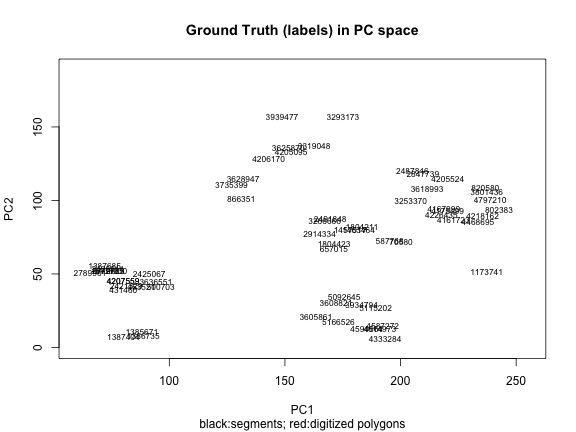``````plot(grtruthDN_meanPCA@data[,c(5,6)],type="n")
text(grtruthDN_meanPCA@data[,c(5,6)],labels=grtruthDN_meanPCA@data\$class,
cex=0.75,col="red")
text(grthsegMSPCAv2@data[,c(6,7)],label=grthsegMSPCAv2@data\$class,
cex=0.75)
title(main="Ground Truth in PC space",
sub="black:segments; red:digitized polygons")
``````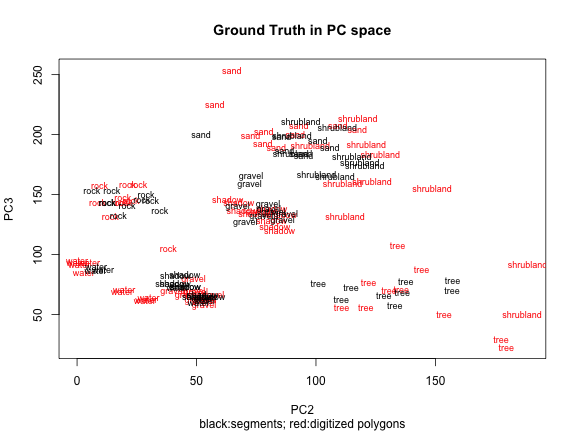``````#Classes are better separated with the revised version (v2)
# errors in water: some "water" segments are actually "shadow"
# revise shrubland and sand again: it might be possible to separate these 2 classes
``````

## 3. Compare unsupervised clusters to ground truth

``````colorCodes <- c("red","green","blue","cyan","yellow","pink","orange",
"black","darkred")
``````

### 3.1 NIR vs RED

``````#TODO
``````

### 3.2 PC2 vs PC1

``````colorines <- mapvalues(hclass9,from=unique(hclass9),to=colorCodes)
plot(grtruthDN_meanPCA@data[,4:5],type="n")
text(segMSPCArnd@data[,3:4],label=hclass9,cex=0.5,
col=alpha(colorines,0.75))
# text(grtruthDN_meanPCA@data[,4:5],label=grtruthDN_meanPCA@data\$class,
#      col="red", cex=0.75)
text(grthsegMSPCAv2@data[,5:6],label=grthsegMSPCAv2@data\$class,cex=0.75)
title(main="Ground Truth in PC2 vs PC1 plane",
sub="black:segments; red:digitized polygons")
``````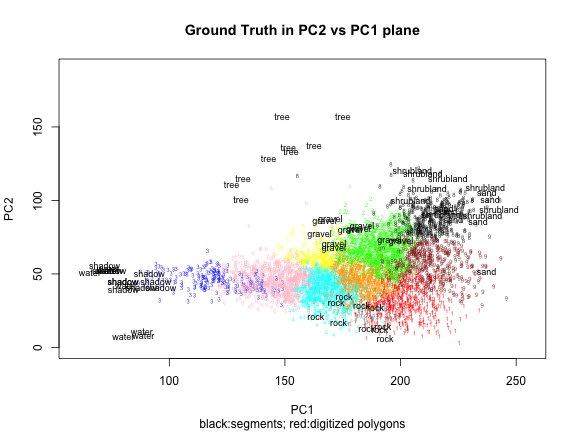## 4. Compare clusters (with repetition) to ground truth

``````colorCodes7 = c("red","green","blue","cyan","yellow","pink","orange")
``````

### 4.1 NIR vs RED

``````#TODO
``````

### 4.2 PC2 vs PC1

``````#colorines7 <- mapvalues(hclass7r,from=unique(hclass7r),to=colorCodes7)
colorCodes9 <- c("red","green","blue","cyan","yellow","pink","orange","black",
"darkred")
colorines9 <- mapvalues(hclass9r,from=unique(hclass9r),to=colorCodes9)
plot(grtruthDN_meanPCA@data[,4:5],type="n")
text(segMSPCArndr@data[,3:4],label=hclass9r,cex=0.7,
col=alpha(colorines9,0.75))
#text(grtruthDN_meanPCA@data[,4:5],label=grtruthDN_meanPCA@data\$grtruthclass,
#     col="red",cex=0.75)
text(grthsegMSPCAv2@data[,5:6],label=grthsegMSPCAv2@data\$class, cex=0.75)
title(main="Ground Truth in PC2 vs PC1 plane",
sub="black:segments; red:digitized polygons")
``````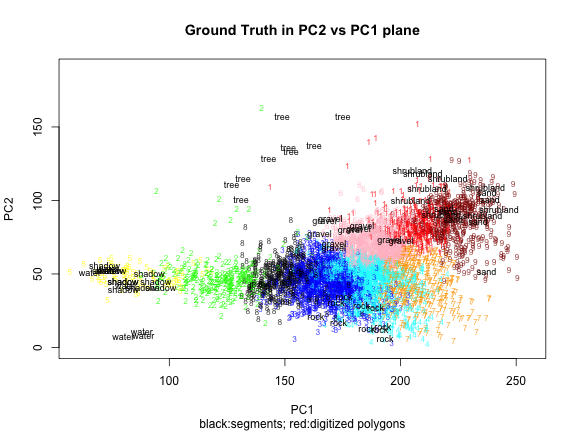## 5. Compare km (with repetition) to ground truth

``````colorCodes = c("red","green","blue","cyan","yellow","pink","orange")
``````

### 5.1 NIR vs RED

``````#TODO
``````

### 5.2 PC2 vs PC1

``````# colorines7 <- mapvalues(segMSPCArndr@data\$km7r,
#                        from=unique(segMSPCArndr@data\$km7r),
#                        to=colorCodes7)
colorCodes9 <- c("red","green","blue","cyan","yellow","pink","orange","black",
"darkred")
colorineskm9r <- mapvalues(km9r\$cluster,from=unique(km9r\$cluster),to=colorCodes9)
plot(grtruthDN_meanPCA@data[,4:5],type="n")
text(segMSPCArndr@data[,3:4],label=segMSPCArndr@data\$km9r,cex=0.7,
col=alpha(colorineskm9r,0.75))
# text(grtruthDN_meanPCA@data[,4:5],label=grtruthDN_meanPCA@data\$class,
#      col="red",cex=0.75)
text(grthsegMSPCAv2@data[,5:6],label=grthsegMSPCAv2@data\$class, cex=0.75)
title(main="Ground Truth and km9r in PC2 vs PC1 plane",
sub="black:segments; red:digitized polygons")
``````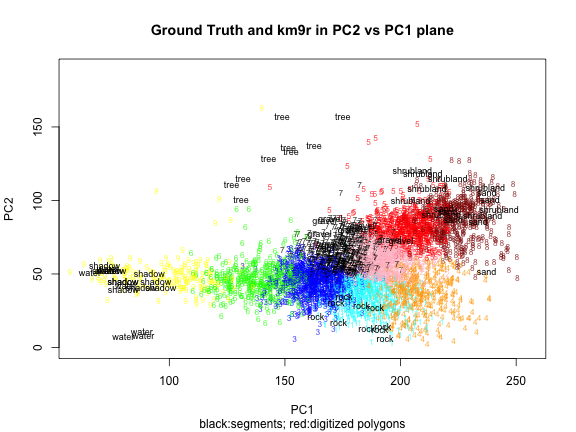## 6. MCLUST

``````#TODO
``````